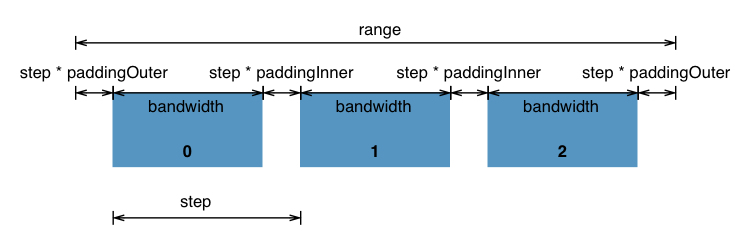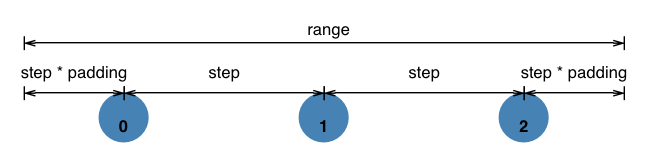# # d3-scale

## # Installing

`NPM` 安装：`npm install d3-scale`. 此外还可以下载 latest release (opens new window). 可以直接从 d3js.org (opens new window)standalone library (opens new window) 或作为 D3 (opens new window) 的一部分直接引入。支持 `AMD`, `CommonJS` 以及基本的标签引入形式。如果使用标签引入则会暴露全局 `d3` 变量：

``````<script src="https://d3js.org/d3-array.v1.min.js"></script>
<script src="https://d3js.org/d3-color.v1.min.js"></script>
<script src="https://d3js.org/d3-format.v1.min.js"></script>
<script src="https://d3js.org/d3-interpolate.v1.min.js"></script>
<script src="https://d3js.org/d3-time.v1.min.js"></script>
<script src="https://d3js.org/d3-time-format.v2.min.js"></script>
<script src="https://d3js.org/d3-scale.v2.min.js"></script>
<script>

var x = d3.scaleLinear();

</script>
``````

(如果不使用 d3.scaleTime or d3.scaleUtc 则可以忽略`d3-time``d3-time-format`.)

## # Continuous Scales

# continuous(value) <> (opens new window)

``````var x = d3.scaleLinear()
.domain([10, 130])
.range([0, 960]);

x(20); // 80
x(50); // 320
``````

``````var color = d3.scaleLinear()
.domain([10, 100])
.range(["brown", "steelblue"]);

color(20); // "#9a3439"
color(50); // "#7b5167"
``````

# continuous.invert(value) <> (opens new window)

``````var x = d3.scaleLinear()
.domain([10, 130])
.range([0, 960]);

x.invert(80); // 20
x.invert(320); // 50
``````

# continuous.domain([domain]) <> (opens new window)

``````var color = d3.scaleLinear()
.domain([-1, 0, 1])
.range(["red", "white", "green"]);

color(-0.5); // "rgb(255, 128, 128)"
color(+0.5); // "rgb(128, 192, 128)"
``````

# continuous.range([range]) <> (opens new window)

# continuous.rangeRound([range]) <> (opens new window)

``````continuous
.range(range)
.interpolate(d3.interpolateRound);
``````

# continuous.clamp(clamp) <> (opens new window)

``````var x = d3.scaleLinear()
.domain([10, 130])
.range([0, 960]);

x(-10); // -160, outside range
x.invert(-160); // -10, outside domain

x.clamp(true);
x(-10); // 0, clamped to range
x.invert(-160); // 10, clamped to domain
``````

# continuous.interpolate(interpolate) <> (opens new window)

If interpolate is specified, sets the scale’s range interpolator factory. This interpolator factory is used to create interpolators for each adjacent pair of values from the range; these interpolators then map a normalized domain parameter t in [0, 1] to the corresponding value in the range. If factory is not specified, returns the scale’s current interpolator factory, which defaults to interpolate (opens new window). See d3-interpolate (opens new window) for more interpolators.

``````var color = d3.scaleLinear()
.domain([-100, 0, +100])
.range(["red", "white", "green"]);
``````

``````var i0 = d3.interpolate("red", "white"),
i1 = d3.interpolate("white", "green");
``````

``````var color = d3.scaleLinear()
.domain([10, 100])
.range(["brown", "steelblue"])
.interpolate(d3.interpolateHcl);
``````

``````var color = d3.scaleLinear()
.domain([10, 100])
.range(["brown", "steelblue"])
.interpolate(d3.interpolateCubehelix.gamma(3));
``````

# continuous.ticks([count])

# continuous.tickFormat([count[, specifier]]) <> (opens new window)

Returns a number format (opens new window) function suitable for displaying a tick value, automatically computing the appropriate precision based on the fixed interval between tick values. The specified count should have the same value as the count that is used to generate the tick values.

``````var x = d3.scaleLinear()
.domain([-1, 1])
.range([0, 960]);

var ticks = x.ticks(5),
tickFormat = x.tickFormat(5, "+%");

ticks.map(tickFormat); // ["-100%", "-50%", "+0%", "+50%", "+100%"]
``````

# continuous.nice([count]) <> (opens new window)

# continuous.copy() <> (opens new window)

### # Linear Scales

# d3.scaleLinear() <> (opens new window)

### # Power Scales

# d3.scalePow() <> (opens new window)

# pow(value) <> (opens new window)

# pow.invert(value) <> (opens new window)

# pow.exponent([exponent]) <> (opens new window)

# pow.domain([domain]) <> (opens new window)

# pow.range([range]) <> (opens new window)

# pow.rangeRound([range]) <> (opens new window)

# pow.clamp(clamp) <> (opens new window)

# pow.interpolate(interpolate) <> (opens new window)

# pow.ticks([count]) <> (opens new window)

# pow.tickFormat([count[, specifier]]) <> (opens new window)

# pow.nice([count]) <> (opens new window)

# pow.copy() <> (opens new window)

# d3.scaleSqrt() <> (opens new window)

### # Log Scales

# d3.scaleLog() <> (opens new window)

# log(value) <> (opens new window)

# log.invert(value) <> (opens new window)

# log.base([base]) <> (opens new window)

If base is specified, sets the base for this logarithmic scale to the specified value. If base is not specified, returns the current base, which defaults to 10.

# log.domain([domain]) <> (opens new window)

# log.range([range]) <> (opens new window)

# log.rangeRound([range]) <> (opens new window)

# log.clamp(clamp) <> (opens new window)

# log.interpolate(interpolate) <> (opens new window)

# log.ticks([count]) <> (opens new window)

continuous.ticks 类似，但是是对数比例尺特定的。如果 base 为整数，则返回的 `ticks` 在基的每个整数幂内间隔均匀。否则，基的每个整数次幂一个 `tick`。如果没有指定 count 则默认为 `10`.

# log.tickFormat([count[, specifier]]) <> (opens new window)

continuous.tickFormat 类似，但是为对数比例尺定特定的。指定的 count 通常与生成 tick values 的个数一致。如果个数太多的话，则 `formatter` 可能会对某些刻度返回空字符串，但是注意刻度仍然会显示。若要禁用筛选，请指定 count 为无穷大。可能还需要一个格式化 specifier 或者格式化函数。例如显示格式为货币并且刻度个数为 `20` 则可以定义为：`log.tickFormat(20, "\$,f")`。如果格式化说明符没有指定精度则会自动计算。

# log.nice() <> (opens new window)

continuous.nice 类似，但是其实现是将 `domain` 扩展为 base 的整数次幂。例如对于输入域为 [0.201479…, 0.996679…], 基为 `10` 的比例尺，则 `domain` 会被扩展为 [0.1, 1]。如果 `domain` 有两个以上元素，则只对第一个和最后一个元素有效。

# log.copy() <> (opens new window)

### # Identity Scales

# d3.scaleIdentity() <> (opens new window)

### # Time Scales

``````var x = d3.scaleTime()
.domain([new Date(2000, 0, 1), new Date(2000, 0, 2)])
.range([0, 960]);

x(new Date(2000, 0, 1,  5)); // 200
x(new Date(2000, 0, 1, 16)); // 640
x.invert(200); // Sat Jan 01 2000 05:00:00 GMT-0800 (PST)
x.invert(640); // Sat Jan 01 2000 16:00:00 GMT-0800 (PST)
``````

# d3.scaleTime() <> (opens new window)

# time(value) <> (opens new window)

# time.invert(value) <> (opens new window)

# time.domain([domain]) <> (opens new window)

# time.range([range]) <> (opens new window)

# time.rangeRound([range]) <> (opens new window)

# time.clamp(clamp) <> (opens new window)

# time.interpolate(interpolate) <> (opens new window)

# time.ticks([count]) <> (opens new window)
# time.ticks([interval])

``````var x = d3.scaleTime();

x.ticks(10);
// [Sat Jan 01 2000 00:00:00 GMT-0800 (PST),
//  Sat Jan 01 2000 03:00:00 GMT-0800 (PST),
//  Sat Jan 01 2000 06:00:00 GMT-0800 (PST),
//  Sat Jan 01 2000 09:00:00 GMT-0800 (PST),
//  Sat Jan 01 2000 12:00:00 GMT-0800 (PST),
//  Sat Jan 01 2000 15:00:00 GMT-0800 (PST),
//  Sat Jan 01 2000 18:00:00 GMT-0800 (PST),
//  Sat Jan 01 2000 21:00:00 GMT-0800 (PST),
//  Sun Jan 02 2000 00:00:00 GMT-0800 (PST)]
``````

• 1-, 5-, 15- and 30-second.
• 1-, 5-, 15- and 30-minute.
• 1-, 3-, 6- and 12-hour.
• 1- and 2-day.
• 1-week.
• 1- and 3-month.
• 1-year.

``````var x = d3.scaleTime()
.domain([new Date(2000, 0, 1, 0), new Date(2000, 0, 1, 2)]);

x.ticks(d3.timeMinute.every(15));
// [Sat Jan 01 2000 00:00:00 GMT-0800 (PST),
//  Sat Jan 01 2000 00:15:00 GMT-0800 (PST),
//  Sat Jan 01 2000 00:30:00 GMT-0800 (PST),
//  Sat Jan 01 2000 00:45:00 GMT-0800 (PST),
//  Sat Jan 01 2000 01:00:00 GMT-0800 (PST),
//  Sat Jan 01 2000 01:15:00 GMT-0800 (PST),
//  Sat Jan 01 2000 01:30:00 GMT-0800 (PST),
//  Sat Jan 01 2000 01:45:00 GMT-0800 (PST),
//  Sat Jan 01 2000 02:00:00 GMT-0800 (PST)]
``````

``````x.ticks(d3.timeMinute.filter(function(d) {
return d.getMinutes() % 15 === 0;
}));
``````

# time.tickFormat([count[, specifier]]) <> (opens new window)
# time.tickFormat([interval[, specifier]])

• `%Y` - 年份，比如 `2011`.
• `%B` - 月份，比如 `February`.
• `%b %d` - 周, 比如 `Feb 06`.
• `%a %d` - 天, 比如 `Mon 07`.
• `%I %p` - 时, 比如 `01 AM`.
• `%I:%M` - 分钟, 比如 `01:23`.
• `:%S` - 秒, 比如 `:45`.
• `.%L` - 毫秒, 比如 `.012`.

# time.nice([count]) <> (opens new window)
# time.nice([interval[, step]])

# d3.scaleUtc() <> (opens new window)

## # Sequential Scales

# d3.scaleSequential(interpolator) <> (opens new window)

``````var rainbow = d3.scaleSequential(function(t) {
return d3.hsl(t * 360, 1, 0.5) + "";
});
``````

``````var rainbow = d3.scaleSequential(d3.interpolateRainbow);
``````

# sequential(value) <> (opens new window)

# sequential.domain([domain]) <> (opens new window)

# sequential.clamp([clamp]) <> (opens new window)

# sequential.interpolator([interpolator]) <> (opens new window)

# sequential.copy() <> (opens new window)

## # Diverging Scales

# d3.scaleDiverging(interpolator) <> (opens new window)

``````var spectral = d3.scaleDiverging(d3.interpolateSpectral);
``````

# diverging(value) <> (opens new window)

# diverging.domain([domain]) <> (opens new window)

# diverging.clamp([clamp]) <> (opens new window)

# diverging.interpolator([interpolator]) <> (opens new window)

# diverging.copy() <> (opens new window)

## # Quantize Scales

# d3.scaleQuantize() <> (opens new window)

# quantize(value) <> (opens new window)

``````var color = d3.scaleQuantize()
.domain([0, 1])
.range(["brown", "steelblue"]);

color(0.49); // "brown"
color(0.51); // "steelblue"
``````

``````var width = d3.scaleQuantize()
.domain([10, 100])
.range([1, 2, 4]);

width(20); // 1
width(50); // 2
width(80); // 4
``````

# quantize.invertExtent(value) <> (opens new window)

``````var width = d3.scaleQuantize()
.domain([10, 100])
.range([1, 2, 4]);

width.invertExtent(2); // [40, 70]
``````

# quantize.domain([domain]) <> (opens new window)

# quantize.range([range]) <> (opens new window)

# quantize.ticks([count]) <> (opens new window)

# quantize.tickFormat([count[, specifier]]) <> (opens new window)

# quantize.nice() <> (opens new window)

# quantize.copy() <> (opens new window)

## # Quantile Scales

# d3.scaleQuantile() <> (opens new window)

# quantile(value) <> (opens new window)

# quantile.invertExtent(value) <> (opens new window)

# quantile.domain([domain]) <> (opens new window)

# quantile.range([range]) <> (opens new window)

# quantile.quantiles() <> (opens new window)

# quantile.copy() <> (opens new window)

## # Threshold Scales

# d3.scaleThreshold() <> (opens new window)

# threshold(value) <> (opens new window)

``````var color = d3.scaleThreshold()
.domain([0, 1])
.range(["red", "white", "green"]);

color(-1);   // "red"
color(0);    // "white"
color(0.5);  // "white"
color(1);    // "green"
color(1000); // "green"
``````

# threshold.invertExtent(value) <> (opens new window)

``````var color = d3.scaleThreshold()
.domain([0, 1])
.range(["red", "white", "green"]);

color.invertExtent("red"); // [undefined, 0]
color.invertExtent("white"); // [0, 1]
color.invertExtent("green"); // [1, undefined]
``````

# threshold.domain([domain]) <> (opens new window)

# threshold.range([range]) <> (opens new window)

# threshold.copy() <> (opens new window)

## # Ordinal Scales

continuous scales 不同，序数比例尺的输出域和输入域都是离散的。例如序数比例尺可以将一组命名类别映射到一组颜色。或者确定一组条形图在水平方向的位置等等。

# d3.scaleOrdinal([range]) <> (opens new window)

# ordinal(value) <> (opens new window)

# ordinal.domain([domain]) <> (opens new window)

# ordinal.range([range]) <> (opens new window)

# ordinal.unknown([value]) <> (opens new window)

# ordinal.copy() <> (opens new window)

# d3.scaleImplicit <> (opens new window)

### # Band Scales# d3.scaleBand() <> (opens new window)

# band(value) <> (opens new window)

# band.domain([domain]) <> (opens new window)

# band.range([range]) <> (opens new window)

# band.rangeRound([range]) <> (opens new window)

``````band
.range(range)
.round(true);
``````

# band.round([round]) <> (opens new window)

# band.align([align]) <> (opens new window)

# band.bandwidth() <> (opens new window)

# band.step() <> (opens new window)

# band.copy() <> (opens new window)

### # Point Scales# d3.scalePoint() <> (opens new window)

# point(value) <> (opens new window)

# point.domain([domain]) <> (opens new window)

# point.range([range]) <> (opens new window)

# point.rangeRound([range]) <> (opens new window)

``````point
.range(range)
.round(true);
``````

# point.round([round]) <> (opens new window)

# point.align([align]) <> (opens new window)

# point.bandwidth() <> (opens new window)

# point.step() <> (opens new window)

# point.copy() <> (opens new window)